# 5 Genetic Algorithm Applications Using PyGAD

3 years ago   •   13 min read

This tutorial introduces PyGAD, an open-source Python library for implementing the genetic algorithm and training machine learning algorithms. PyGAD supports 19 parameters for customizing the genetic algorithm for various applications.

Within this tutorial we'll discuss 5 different applications of the genetic algorithm and build them using PyGAD.

The outline of the tutorial is as follows:

• Fitting a Linear Model
• Reproducing Images
• 8 Queen Puzzle
• Training Neural Networks
• Training Convolutional Neural Networks

You can follow along with each of these projects and run them for free on the ML Showcase. Let's get started.

Bring this project to life

PyGAD is available through PyPI (Python Package Index) and thus it can be installed simply using pip. For Windows, simply use the following command:

pip install pygad

For Mac/Linux, use pip3 instead of pip in the terminal command:

pip3 install pygad

Then make sure the library is installed by importing it from the Python shell:

python
import pygad

The latest PyGAD version is currently 2.3.2, which was released on June 1st 2020. Using the __version__ special variable, the current version can be returned.

import pygad

print(pygad.__version__)

Now that PyGAD is installed, let's cover a brief introduction to PyGAD.

The main goal of PyGAD is to provide a simple implementation of the genetic algorithm. It offers a range of parameters that allow the user to customize the genetic algorithm for a wide range of applications. Five such applications are discussed in this tutorial.

The full documentation of PyGAD is available at Read the Docs. Here we'll cover a more digestible breakdown of the library.

In PyGAD 2.3.2 there are 5 modules:

1. pygad: The main module comes already imported.
2. pygad.nn: For implementing neural networks.
3. pygad.gann: For training neural networks using the genetic algorithm.
4. pygad.cnn: For implementing convolutional neural networks.
5. pygad.gacnn: For training convolutional neural networks using the genetic algorithm.

Each module has its own repository on GitHub, linked below.

The main module of the library is named pygad. This module has a single class named GA. Just create an instance of the pygad.GA class to use the genetic algorithm.

The steps to use the pygad module are:

1. Create the fitness function.
2. Prepare the necessary parameters for the pygad.GA class.
3. Create an instance of the pygad.GA class.
4. Run the genetic algorithm.

In PyGAD 2.3.2, the constructor of the pygad.GA class has 19 parameters, of which 16 are optional. The three required parameters are:

1. num_generations: Number of generations.
2. num_parents_mating: Number of solutions to be selected as parents.
3. fitness_func: The fitness function that calculates the fitness value for the solutions.

The fitness_func parameter is what allows the genetic algorithm to be customized for different problems. This parameter accepts a user-defined function that calculates the fitness value for a single solution. This takes two additional parameters: the solution, and its index within the population.

Let's see an example to make this clearer. Assume there is a population with 3 solutions, as given below.

[221, 342, 213]
[675, 32, 242]
[452, 23, -212]

The assigned function to the fitness_func parameter must return a single number representing the fitness of each solution. Here is an example that returns the sum of the solution.

def fitness_function(solution, solution_idx):
return sum(solution)

The fitness values for the 3 solutions are then:

1. 776
2. 949
3. 263

The parents are selected based on such fitness values. The higher the fitness value, the better the solution.

For the complete list of parameters in the pygad.GA class constructor, check out this page.

After creating an instance of the pygad.GA class, the next step is to call the run() method which goes through the generations that evolve the solutions.

import pygad

ga_instance.run()

These are the essential steps for using PyGAD. Of course there are additional steps that can be taken as well, but this is the minimum needed.

The next sections discuss using PyGAD for several different use cases.

## Fitting a Linear Model

Assume there is an equation with 6 inputs, 1 output, and 6 parameters, as follows:

 y = f(w1:w6) = w1x1 + w2x2 + w3x3 + w4x4 + w5x5 + 6wx6

Let's assume that the inputs are (4,-2,3.5,5,-11,-4.7) and the output is 44. What are the values for the 6 parameters to satisfy the equation? The genetic algorithm can be used to find the answer.

The first thing to do is to prepare the fitness function as given below. It calculates the sum of products between each input and its corresponding parameter. The absolute difference between the desired output and the sum of products is calculated. Because the fitness function must be a maximization function, the returned fitness is equal to 1.0/difference. The solutions with the highest fitness values are selected as parents.

function_inputs = [4,-2,3.5,5,-11,-4.7]  # Function inputs.
desired_output = 44  # Function output.

def fitness_func(solution, solution_idx):
output = numpy.sum(solution*function_inputs)
fitness = 1.0 / numpy.abs(output - desired_output)
return fitness

Now that we've prepared the fitness function, here's a list with other important parameters.

sol_per_pop = 50
num_genes = len(function_inputs)

init_range_low = -2
init_range_high = 5

mutation_percent_genes = 1

You should also specify your desired mandatory parameters as you see fit. After the necessary parameters are prepared, the pygad.GA class is instantiated. For information about each of the parameters, refer to this page.

ga_instance = pygad.GA(num_generations=num_generations,
num_parents_mating=num_parents_mating,
fitness_func=fitness_func,
sol_per_pop=sol_per_pop,
num_genes=num_genes,
init_range_low=init_range_low,
init_range_high=init_range_high,
mutation_percent_genes=mutation_percent_genes)

The next step is to call the run() method which starts the generations.

ga_instance.run()

After the run() method completes, the plot_result() method can be used to show the fitness values over the generations.

ga_instance.plot_result()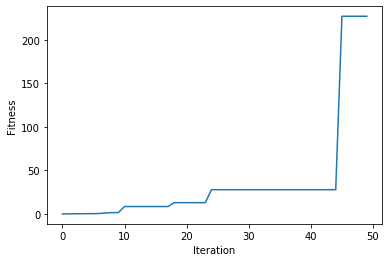Using the best_solution() method we can also retrieve what the best solution was, its fitness, and its index within the population.

solution, solution_fitness, solution_idx = ga_instance.best_solution()
print("Parameters of the best solution : {solution}".format(solution=solution))
print("Fitness value of the best solution = {solution_fitness}".format(solution_fitness=solution_fitness))
print("Index of the best solution : {solution_idx}".format(solution_idx=solution_idx))

The full code for this project can be found in the Fitting a Linear Model notebook on the ML Showcase.

## Reproducing Images

In this application we'll start from a random image (random pixel values), then evolve the value of each pixel using the genetic algorithm.

The tricky part of this application is that an image is 2D or 3D, and the genetic algorithm expects the solutions to be 1D vectors. To tackle this issue we'll use the img2chromosome() function defined below to convert an image to a 1D vector.

def img2chromosome(img_arr):

return numpy.reshape(a=img_arr, newshape=(functools.reduce(operator.mul, img_arr.shape)))

The chromosome2img() function (below) can then be used to restore the 2D or 3D image back from the vector.

def chromosome2img(vector, shape):
# Check if the vector can be reshaped according to the specified shape.
if len(vector) != functools.reduce(operator.mul, shape):
raise ValueError("A vector of length {vector_length} into an array of shape {shape}.".format(vector_length=len(vector), shape=shape))

return numpy.reshape(a=vector, newshape=shape)

import imageio
import numpy

target_im = numpy.asarray(target_im/255, dtype=numpy.float)

Next, the fitness function is prepared. This will calculate the difference between the pixels in the solution and the target images. To make it a maximization function, the difference is subtracted from the sum of all pixels in the target image.

target_chromosome = gari.img2chromosome(target_im)

def fitness_fun(solution, solution_idx):
fitness = numpy.sum(numpy.abs(target_chromosome-solution))

# Negating the fitness value to make it increasing rather than decreasing.
fitness = numpy.sum(target_chromosome) - fitness
return fitness

The next step is to create an instance of the pygad.GA class, as shown below. It is critical to the success of the application to use appropriate parameters. If the range of pixel values in the target image is 0 to 255, then the init_range_low and init_range_high must be set to 0 and 255, respectively. The reason is to initialize the population with images of the same data type as the target image. If the image pixel values range from 0 to 1, then the two parameters must be set to 0 and 1, respectively.

import pygad

num_parents_mating=10,
fitness_func=fitness_fun,
sol_per_pop=20,
num_genes=target_im.size,
init_range_low=0.0,
init_range_high=1.0,
mutation_percent_genes=0.01,
mutation_type="random",
mutation_by_replacement=True,
random_mutation_min_val=0.0,
random_mutation_max_val=1.0)

When the mutation_type argument is set to random, then the default behavior is to add a random value to each gene selected for mutation. This random value is selected from the range specified by the random_mutation_min_val and random_mutation_max_val parameters.

Assume the range of pixel values is 0 to 1. If a pixel has the value 0.9 and a random value of 0.3 is generated, then the new pixel value is 1.2. Because the pixel values must fall within the 0 to 1 range, the new pixel value is therefore invalid. To work around this issue, it is very important to set the mutation_by_replacement parameter to True. This causes the random value to replace the current pixel rather than being added to the pixel.

After the parameters are prepared, then the genetic algorithm can run.

ga_instance.run()

The plot_result() method can be used to show how the fitness value evolves by generation.

ga_instance.plot_result()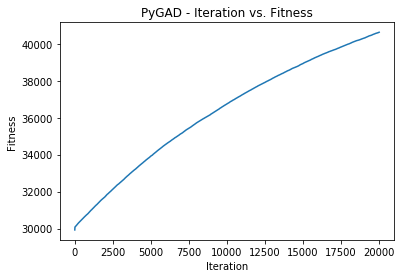After the generations complete, some information can be returned about the best solution.

solution, solution_fitness, solution_idx = ga_instance.best_solution()
print("Fitness value of the best solution = {solution_fitness}".format(solution_fitness=solution_fitness))
print("Index of the best solution : {solution_idx}".format(solution_idx=solution_idx))

The best solution can be converted into an image to be displayed.

import matplotlib.pyplot

result = gari.chromosome2img(solution, target_im.shape)
matplotlib.pyplot.imshow(result)
matplotlib.pyplot.show()

Here is the result.## 8 Queen Puzzle

The 8 Queen Puzzle involves 8 chess queens distributed across an 8×8 matrix, with one queen per row. The goal is to place these queens such that no queen can attack another one vertically, horizontally, or diagonally. The genetic algorithm can be used to find a solution that satisfies such conditions.

This project is available on GitHub. It has a GUI built using Kivy that shows an 8×8 matrix, as shown in the next figure.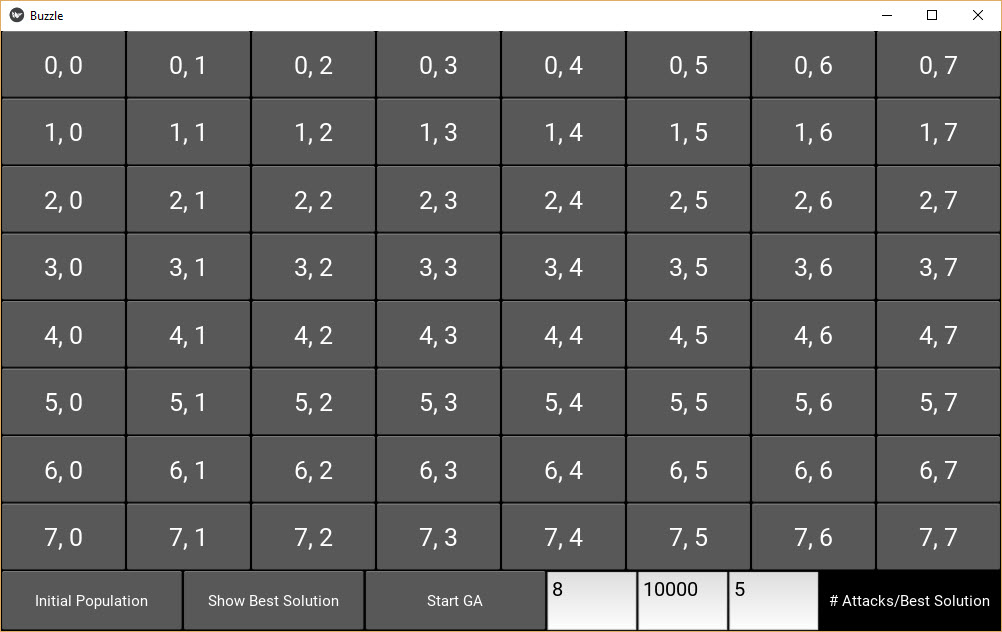The GUI has three buttons at the bottom of the screen. The function of these buttons are as follows:

• The Initial Population button creates the initial population of the GA.
• The Show Best Solution button shows the best solution from the last generation the GA stopped at.
• The Start GA button starts the GA iterations/generations.

To use this project start by pressing the Initial Population button, followed by the Start GA button. Below is the method called by the Initial Population button which, as you might have guessed, generates the initial population.

def initialize_population(self, *args):
self.num_solutions = 10

self.reset_board_text()

self.population_1D_vector = numpy.zeros(shape=(self.num_solutions, 8))

for solution_idx in range(self.num_solutions):
initial_queens_y_indices = numpy.random.rand(8)*8
initial_queens_y_indices = initial_queens_y_indices.astype(numpy.uint8)
self.population_1D_vector[solution_idx, :] = initial_queens_y_indices

self.vector_to_matrix()

self.pop_created = 1
self.num_attacks_Label.text = "Initial Population Created."

Each solution in the population is a vector with 8 elements referring to the column indices of the 8 queens. To show the queens' locations on the screen, the 1D vector is converted into a 2D matrix using the vector_to_matrix() method. The next figure shows the queens on the screen.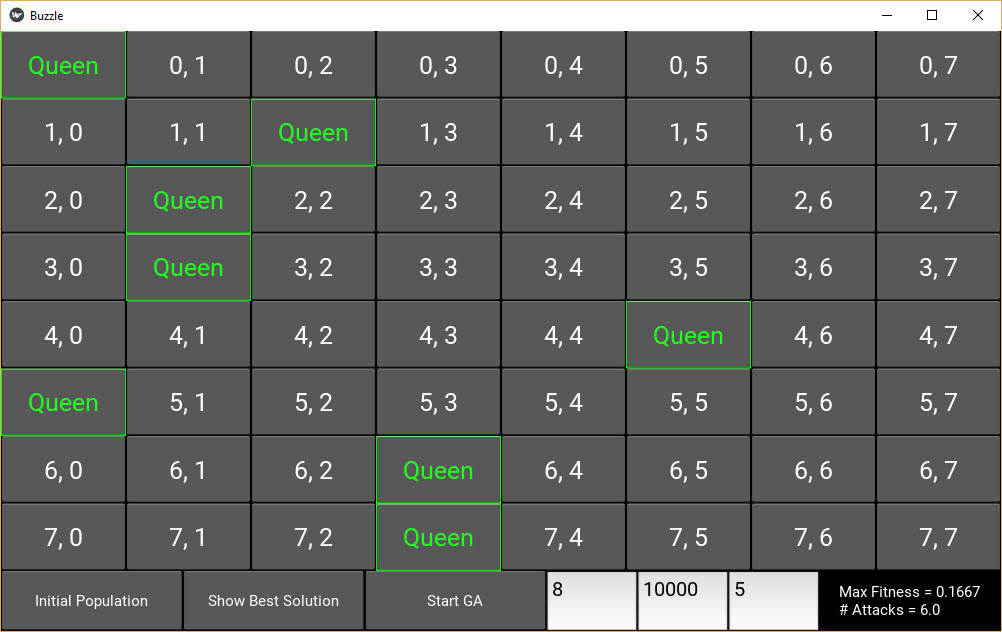Now that the GUI is built, we'll build and run the genetic algorithm using PyGAD.

The fitness function used in this project is given below. It simply calculates the number of attacks that can be made by each of the 8 queens and returns this as the fitness value.

def fitness(solution_vector, solution_idx):

if solution_vector.ndim == 2:
solution = solution_vector
else:
solution = numpy.zeros(shape=(8, 8))

row_idx = 0
for col_idx in solution_vector:
solution[row_idx, int(col_idx)] = 1
row_idx = row_idx + 1

total_num_attacks_column = attacks_column(solution)

total_num_attacks_diagonal = attacks_diagonal(solution)

total_num_attacks = total_num_attacks_column + total_num_attacks_diagonal

if total_num_attacks == 0:
total_num_attacks = 1.1 # float("inf")
else:
total_num_attacks = 1.0/total_num_attacks

return total_num_attacks

By pressing the Start GA button, an instance of the pygad.GA class is created and the run() method is called.

ga_instance = pygad.GA(num_generations=500,
num_parents_mating=5,
fitness_func=fitness,
num_genes=8,
initial_population=self.population_1D_vector,
mutation_percent_genes=0.01,
mutation_type="random",
mutation_num_genes=3,
mutation_by_replacement=True,
random_mutation_min_val=0.0,
random_mutation_max_val=8.0,
callback_generation=callback)

ga_instance.run()

Here is a possible solution in which the 8 queens are placed on the board where no queen attacks another.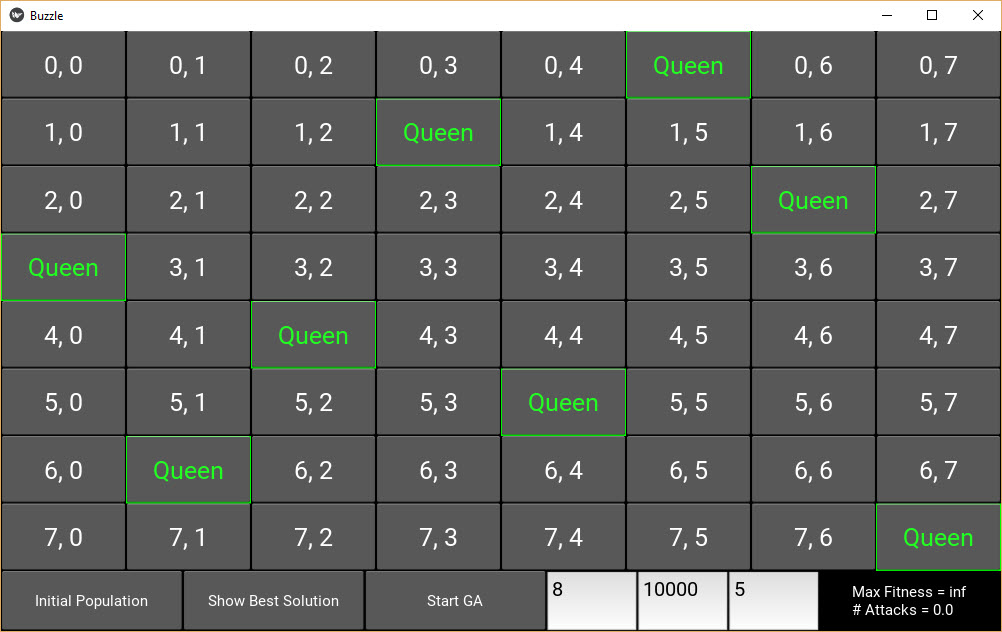The complete code for this project can be found on GitHub.

## Training Neural Networks

Among other types of machine learning algorithms, the genetic algorithm can be used to train neural networks. PyGAD supports training neural networks and, in particular, convolutional neural networks, by using the pygad.gann.GANN and pygad.gacnn.GACNN modules. This section discusses how to use the pygad.gann.GANN module for training neural networks for a classification problem.

Before building the genetic algorithm, the training data is prepared. This example builds a network that simulates the XOR logic gate.

# Preparing the NumPy array of the inputs.
data_inputs = numpy.array([[1, 1],
[1, 0],
[0, 1],
[0, 0]])

# Preparing the NumPy array of the outputs.
data_outputs = numpy.array([0,
1,
1,
0])

The next step is to create an instance of the pygad.gann.GANN class. This class builds a population of neural networks that all have the same architecture.

num_inputs = data_inputs.shape
num_classes = 2

num_solutions = 6
num_neurons_input=num_inputs,
num_neurons_hidden_layers=,
num_neurons_output=num_classes,
hidden_activations=["relu"],
output_activation="softmax")

After creating the instance of the pygad.gann.GANN class, the next step is to create the fitness function. This returns the classification accuracy for the passed solution.

import pygad.nn

def fitness_func(solution, sol_idx):
global GANN_instance, data_inputs, data_outputs

data_inputs=data_inputs)
correct_predictions = numpy.where(predictions == data_outputs).size
solution_fitness = (correct_predictions/data_outputs.size)*100

return solution_fitness

Besides the fitness function, the other necessary parameters are prepared which we discussed previously.

population_vectors = pygad.gann.population_as_vectors(population_networks=GANN_instance.population_networks)

initial_population = population_vectors.copy()

num_parents_mating = 4

num_generations = 500

mutation_percent_genes = 5

parent_selection_type = "sss"

crossover_type = "single_point"

mutation_type = "random"

keep_parents = 1

init_range_low = -2
init_range_high = 5

After all parameters are prepared, an instance of the pygad.GA class is created.

ga_instance = pygad.GA(num_generations=num_generations,
num_parents_mating=num_parents_mating,
initial_population=initial_population,
fitness_func=fitness_func,
mutation_percent_genes=mutation_percent_genes,
init_range_low=init_range_low,
init_range_high=init_range_high,
parent_selection_type=parent_selection_type,
crossover_type=crossover_type,
mutation_type=mutation_type,
keep_parents=keep_parents,
callback_generation=callback_generation)

The callback_generation parameter refers to a function that is called after each generation. In this application, this function is used to update the weights of all the neural networks after each generation.

def callback_generation(ga_instance):
global GANN_instance

GANN_instance.update_population_trained_weights(population_trained_weights=population_matrices)

The next step is to call the run() method.

ga_instance.run()

After the run() method completes, the next figure shows how the fitness value evolved. The figure shows that a classification accuracy of 100% is reached.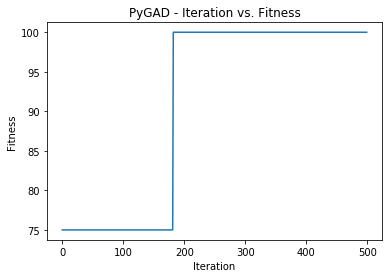The complete code for building and training the neural network can be accessed and run for free on the ML Showcase in the Training Neural Networks notebook.

## Training Convolutional Neural Networks

Similar to training multilayer perceptrons, PyGAD supports training convolutional neural networks using the genetic algorithm.

The first step is to prepare the training data. The data can be downloaded from these links:

1. dataset_inputs.npy: Data inputs.
2. dataset_outputs.npy: Class labels.
import numpy

train_outputs = numpy.load("dataset_outputs.npy")

The next step is to build the CNN architecture using the pygad.cnn module.

import pygad.cnn

kernel_size=3,
previous_layer=input_layer,
activation_function="relu")
previous_layer=conv_layer,
stride=3)

previous_layer=flatten_layer,
activation_function="softmax")

After the layers in the network are stacked, a model is created.

model = pygad.cnn.Model(last_layer=dense_layer,
epochs=5,
learning_rate=0.01)

Using the summary() method, a summary of the model architecture is returned.

----------Network Architecture----------
<class 'cnn.Conv2D'>
<class 'cnn.AveragePooling2D'>
<class 'cnn.Flatten'>
<class 'cnn.Dense'>
----------------------------------------

After the model is prepared, the pygad.gacnn.GACNN class is instantiated to create the initial population. All the networks have the same architecture.

import pygad.gacnn

num_solutions=4)

The next step is to prepare the fitness function. This calculates the classification accuracy for the passed solution.

def fitness_func(solution, sol_idx):
global GACNN_instance, data_inputs, data_outputs

predictions = GACNN_instance.population_networks[sol_idx].predict(data_inputs=data_inputs)
correct_predictions = numpy.where(predictions == data_outputs).size
solution_fitness = (correct_predictions/data_outputs.size)*100

return solution_fitness

The other parameters are also prepared.

population_vectors = pygad.gacnn.population_as_vectors(population_networks=GACNN_instance.population_networks)

initial_population = population_vectors.copy()

num_parents_mating = 2

num_generations = 10

mutation_percent_genes = 0.1

parent_selection_type = "sss"

crossover_type = "single_point"

mutation_type = "random"

keep_parents = -1

After all parameters are prepared, an instance of the pygad.GA class is created.

ga_instance = pygad.GA(num_generations=num_generations,
num_parents_mating=num_parents_mating,
initial_population=initial_population,
fitness_func=fitness_func,
mutation_percent_genes=mutation_percent_genes,
parent_selection_type=parent_selection_type,
crossover_type=crossover_type,
mutation_type=mutation_type,
keep_parents=keep_parents,
callback_generation=callback_generation)

The callback_generation parameter is used to update the network weights after each generation.

def callback_generation(ga_instance):
global GACNN_instance, last_fitness

GACNN_instance.update_population_trained_weights(population_trained_weights=population_matrices)

The last step is to call the run() method.

ga_instance.run()

The complete code for building and training the convolutional neural network can be found on the ML Showcase, where you can also run it on a free GPU from your free Gradient account.

## Conclusion

This tutorial introduced PyGAD, an open-source Python library for implementing the genetic algorithm. The library supports a number of parameters to customize the genetic algorithm for a number of applications.

In this tutorial we used PyGAD to build 5 different applications including fitting a linear model, solving the 8 queens puzzle, reproducing images, and training neural networks (both conventional and convolutional). I hope you found this tutorial useful, and please feel free reach out in the comments or check out the docs if you have any questions!